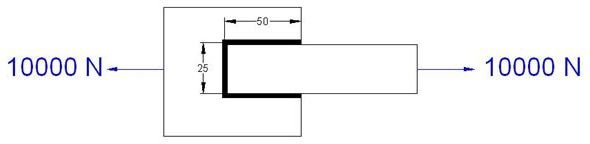# Welding Design Tutorial for Correct Welding Size Calculation and Preparing Welding Drawing for Pure Tensile Loading

Exact welding size calculations may vary based upon the geometry of the welded plates. The example we discuss here will give you an overview, and same methodology can be applied effectively for design calculations of all types of lap weld joints under pure tensile loading.

Please refer to the welding joint picture below. (Click to enlarge.)As a welding design engineer, your task is to find the weld size or leg length based on the given loads and welding rod filler material strength.

## Input Data

Applied force F = 10000 N

Total length of the weld L = 50+50+25 MM= 125 mm

Design stress of the filler material Pw = 220 N/mm2

## Steps for Calculating Welding Size

• Assume throat thickness for the weld as t.
• The total area (A), which is resisting the force F can be calculated as:

A = L*t

=125* t mm2

• Developed shear stress (T) in the weld can be calculated as:

T = F/A

= 10000/(125*t) N/mm2

T =Pw

Or, 10000 / (125*t) = 220

Or, t = 0.36 mm

• Leg length (s) of the weld can be calculated from the following equation:

s = 1.414 * t

= 1.414 * 0.36 mm

= 0.509 mm (0.6 mm approx.)

## Conclusion

The above example explains the steps for calculating welding joint for lap joint and for pure tensile loading conditions. For complex bending and torsion loading line method is used for calculating welding sizes. FEA tools can play important role for calculating correct weld size for extremely complicated joints.